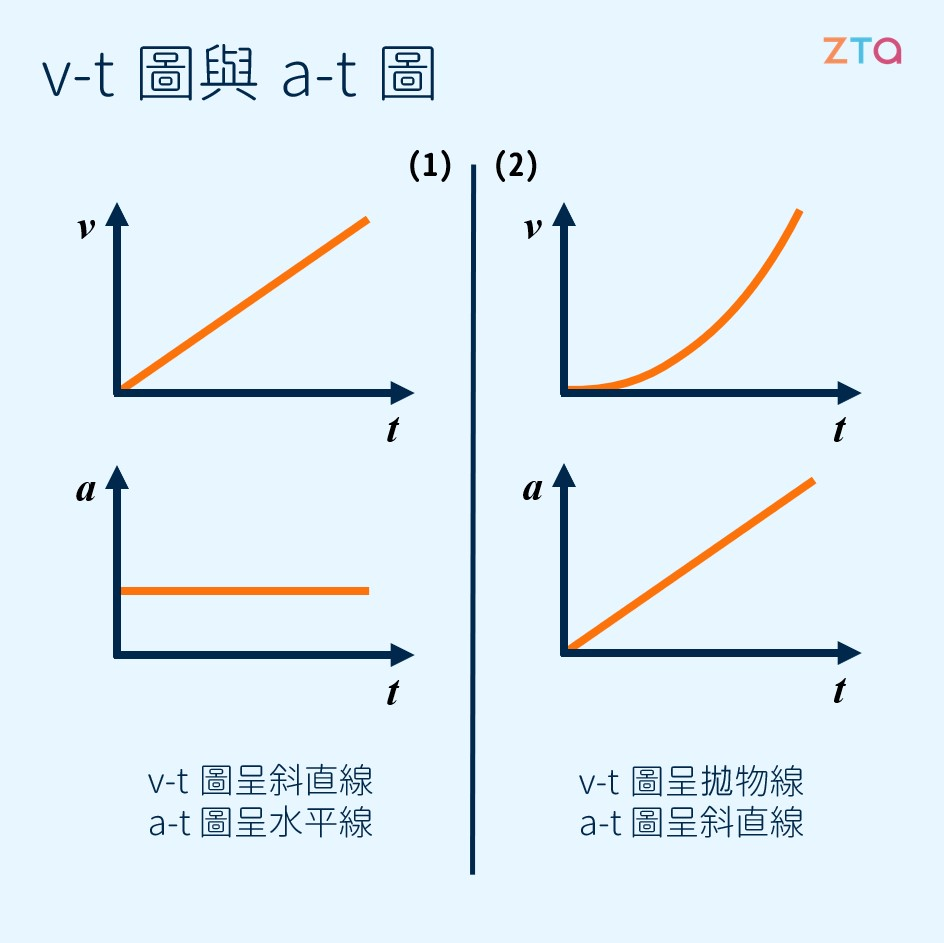# 加速度

## 加速度是速度的變化

$$a = \frac{\Delta v}{t}$$

$$(\frac{m}{s})/s = m/s^2$$

## V-t 圖與 a-t 圖## 等加速度運動

$$\Delta v = a\cdot t$$

+1